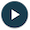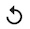# Training and Test Sets: Playground Exercise

## Training Sets and Test Sets

We return to Playground to experiment with training sets and test sets.

This exercise provides both a test set and a training set, both drawn from the same data set. By default, the visualization shows only the training set. If you'd like to also see the test set, click the Show test data checkbox just below the visualization. In the visualization, note the following distinction:

• The training examples have a white outline.
• The test examples have a black outline.

Task 1: Run Playground with the given settings by doing the following:

1. Click the Run/Pause button:2. Watch the Test loss and Training loss values change.
3. When the Test loss and Training loss values stop changing or only change once in a while, press the Run/Pause button again to pause Playground.
Note the delta between the Test loss and Training loss. We'll try to reduce this delta in the following tasks.

Task 2: Do the following:

1. Press the Reset button.2. Modify the Learning rate.
3. Press the Run/Pause button:4. Let Playground run for at least 150 epochs.

Is the delta between Test loss and Training loss lower or higher with this new Learning rate? What happens if you modify both Learning rate and batch size?

Optional Task 3: A slider labeled Training data percentage lets you control the proportion of training data to test data. For example, when set to 90%, then 90% of the data is used for the training set and the remaining 10% is used for the test set.

Do the following:

1. Reduce the "Training data percentage" from 50% to 10%.
2. Experiment with Learning rate and Batch size, taking notes on your findings.
Does altering the training data percentage change the optimal learning settings that you discovered in Task 2? If so, why?

[]
[]